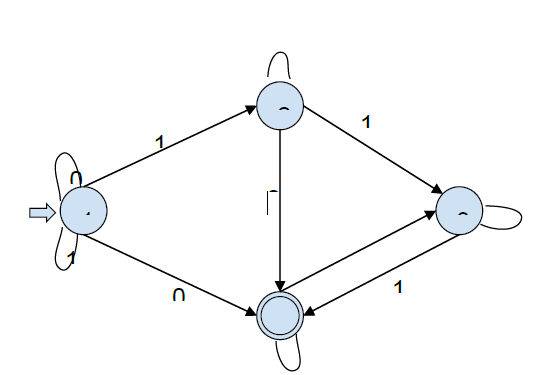# C program to simulate Nondeterministic Finite Automata (NFA)

In this problem, we are will create a C program to simulate non-deterministic Finite automata (NFA).

NFA (Non-deterministic Finite automata) finite state machine that can move to any combination of states for an input symbol i.e. there is no exact state to which the machine will move.

Formal definition of NDFA −

NFA / NDFA (Non-deterministic Finite automata) can be represented by 5-tuple (Q, ∑, δ, q0, F) where −

• Q is a finite set of states.

• ∑ is a finite set of symbols called the alphabets.

• δ is the transition function where d: Q × ∑ → 2Q (Here the power set of Q (2Q) has been taken because in case of NDFA, from a state, transition can occur to any combination of Q states)

• q0 is the initial state from where any input is processed (q0 ∈ Q).

• F is a set of final state/states of Q (F ⊆ Q).

In programming, NFA is created using a directed graph. Each vertex of the graph denotes the states of NDA. The edges of the graph can have one of the two values 0 or 1. Edge labeled as 0 represents non-accepting transition whereas Edge labeled as 1 represents accepting transition.

There is an entry point to the graph generally vertex 1 from where it takes input string which is a binary array of finite length.

Let’s see an NFA graphical form and then solve a grammar using it.Starting state -> 1

Final state (accepting state) -> 4

Let’s check whether string 01001 is accepted or not.

Starting state 1, input 0, with 0 we can go to state 4 or self-loop to state 1.

We will consider both cases −

{1->1} 1001
{1->4} 1001

State 1/4, input 1 −

From state 1, we can go to 2 or self-loop, From state 4, we cannot go further so we will discard this case.

We will consider cases from one −

{1->1->1} 001
{1->1->2} 001

State 1/2, input 0 −

From state 1, we can go to 4 or self-loop,
From state 2, we can go to 4 or self-loop

We will consider all cases −

{1->1->1->1} 01
{1->1->1->4} 01
{1->1->2->1} 01
{1->1->2->4} 01

State 1/2/4, input 0 −

From state 1, we can go to 4 or self-loop,
From state 2, we can go to 4 or self-loop,
From state 4, we can go to 3 or self-loop.

We will consider all cases −

{1->1->1->1->1} 1
{1->1->1->1->4} 1
{1->1->1->4->3} 1
{1->1->1->4->4} 1
{1->1->2->1->1} 1
{1->1->2->1->4} 1
{1->1->2->4->3} 1
{1->1->2->4->4} 1

State 1/2/3/4, input 1 −

From state 1, we can go to 2 or self-loop,
From state 2, we can go to 3,
From state 3, we can go to 4,
From state 4, we cannot go further.

We will consider all cases −

{1->1->1->1->1->1/2} does not reach final stage
{1->1->1->1->4} 1 cannot accept input
{1->1->1->4->3 ->4} accepts the input
{1->1->1->4->4} cannot accept input
{1->1->2->1->1 -> 1/2} does not reach final stage
{1->1->2->1->4} cannot accept input
{1->1->2->4->3->4} accepts the input
{1->1->2->4->4} cannot accept input

Hence there are ways to reach the final state with the given input string.

Now, let’s get to the C program to simulate Nondeterministic Finite Automata (NFA) −

The input of the program will be an adjacency list of NFA −

Number of edges (n)

Edge connectivity (n lines)

Strings to be checked

## Example

4
1031204
21104
301041204
4120114
101101

## Output

Yes/No

## Example

#include <stdio.h>
#include <stdlib.h>
#include <string.h>
#include <stdbool.h>
#include <math.h>
int row = 0;
struct node{
int data;
struct node* next;
char edgetype;
}typedef node;
node* push(node* first , char edgetype , int data){
node* new_node = (node*)malloc(sizeof(node));
new_node->edgetype = edgetype;
new_node->data = data;
new_node->next = NULL;
if (first==NULL){
first = new_node;
return new_node;
}
first->next = push(first->next,edgetype,data);
return first;
}
//Recursive function to check acceptance of input
int nfa(node** graph, int current, char* input,
int* accept, int start){
if (start==(int)strlen(input))
return accept[current];
node* temp = graph[current];
while (temp != NULL){
if (input[start]==temp->edgetype) {
if (nfa(graph,temp->data,input,accept,start+1==1)){
return 1;
}
}
temp=temp->next;
}
return 0;
}
//Function to generate binary strings of size n
void generate(char** arr, int size, char *a){
if (size==0){
strcpy(arr[row], a);
row++;
return;
}
char b0 = {'\0'};
char b1 = {'\0'};
b0 = '0';
b1 = '1';
generate((char**)arr, size-1, strcat(b0,a)); //Add 0 in front
generate((char**)arr, size-1, strcat(b1,a)); //Add 1 in front
return;
}
int main(){
int n;
int i, j;
scanf("%d", &n); //Number of nodes
node* graph[n+1]; //Create a graph
for (i=0;i<n+1;i++)
graph[i]=NULL;
int accept[n+1]; //Array to store state of vertex
for (i=0; i<n; i++){
//Index of vertex , Acceptance state , Number of edges
int index,acc,number_nodes;
scanf("%d%d%d",&index,&acc,&number_nodes);
accept[index]=acc; //Store acceptance
int edge;
}
}
int size = 1; //Size of input
int count = 0; //Keep count of output strings
if (accept==1) //Check for empty string{
printf("e");
count++;
}
while (count < 11){
char** arr;
int power = pow(2,size);
arr = (char**)malloc(power*sizeof(char*));
for (i=0;i<power;i++)
arr[i] = (char*)malloc(size*sizeof(char));
char a = {'\0'};
generate((char**)arr,size,a); //Generate inputs
for (i=0; i<power; i++){
char input = {'\0'};
for (j=0; j<size; j++){
char foo;
foo = arr[i][size-1-j];
foo = '\0';
strcat(input,foo);
//Copy generated string input
}
int result = nfa(graph,1,input,accept,0);
// Store result of nfa
if (result==1){
printf("%s",input);
count++;
}
if (count==10)
return 0;
}
size++; //Increment size of binary string input
row=0;
}
return 0;
}

## Input

4
1 0 4 0 1 0 2 1 1 1 3
2 0 1 0 4
3 0 1 1 4
4 1 2 0 4 1 4

## Output

00
11
000
001
011
100
110
111
0000
0001